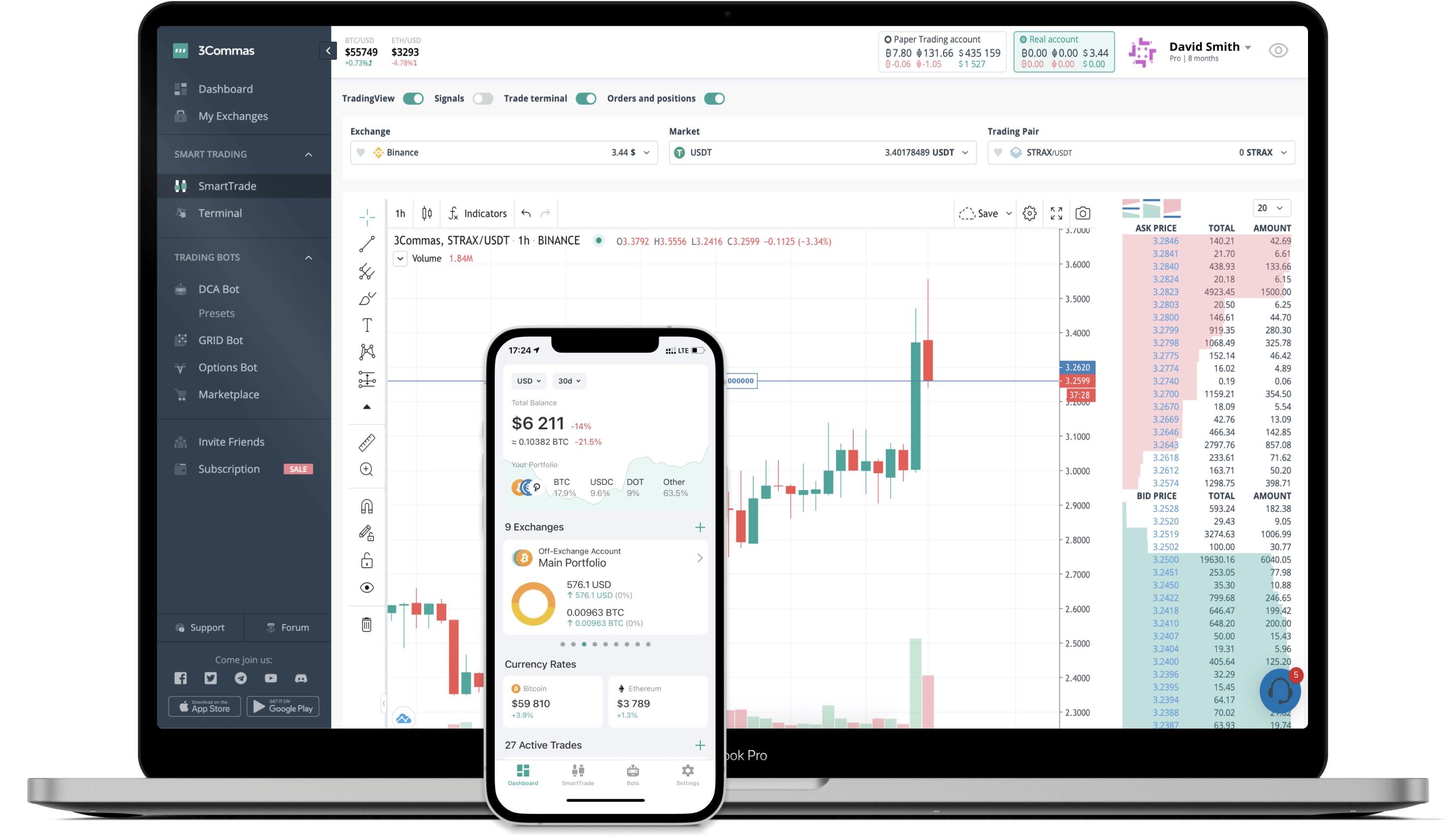# MATH to EUR Converter

MATH
Price0.73-4.80%
Volume832,561
How it works

The 3Commas currency calculator allows you to convert a currency from MATH (MATH) to Euro (EUR) in just a few clicks at live exchange rates.

Simply enter the amount of MATH you wish to convert to EUR and the conversion amount automatically populates. You can also use our Prices Calculator Table to calculate how much your currency is worth in other denominations, i.e. .1 MATH, .5 MATH, 1 MATH, 5 MATH, or even 10 MATH.

## MATH to EUR Exchange Calculator

The chart displays MATH price changes in EUR.

The ultimate tools for crypto traders to maximize trading profits while minimizing risk and loss.## Latest MATH to EUR price calculator

MATH market price is updated every three minutes and is automatically displayed in EUR. Below are the most popular denominations to convert to EUR.

MATH
Euro
0.01
MATH
0.01
EUR
0.1
MATH
0.07
EUR
1
MATH
0.73
EUR
2
MATH
1.47
EUR
3
MATH
2.20
EUR
5
MATH
3.67
EUR
10
MATH
7.33
EUR
25
MATH
18.34
EUR
50
MATH
36.67
EUR
100
MATH
73.34
EUR
250
MATH
183.36
EUR
500
MATH
366.72
EUR
1000
MATH
733.45
EUR
2500
MATH
1833.62
EUR
Euro
MATH
0.01
EUR
0.01363425
MATH
0.1
EUR
0.13634249
MATH
1
EUR
1.36342488
MATH
2
EUR
2.72684975
MATH
3
EUR
4.09027463
MATH
5
EUR
6.81712438
MATH
10
EUR
13.63424875
MATH
25
EUR
34.08562188
MATH
50
EUR
68.17124375
MATH
100
EUR
136.34248751
MATH
250
EUR
340.85621876
MATH
500
EUR
681.71243753
MATH
1000
EUR
1363.42487505
MATH
2500
EUR
3408.56218763
MATH

## FAQ

### How much is 1 MATH in Euro?

MATH price in EUR is constantly changing.

At this moment, 1 MATH equals 0.7334470848355841 EUR

### How do I calculate the conversion from MATH to EUR?

The 3Commas MATH Calculator allows you to easily calculate the conversion price of MATH to EUR by simply entering the amount of MATH in the corresponding field and will automatically convert the value in Euro (EUR).

You can also use our MATH price table above to check the latest MATH price in major fiat and crypto currencies.

### How can I convert MATH to EUR?

The most common way of converting MATH to EUR is by using a Crypto Exchange or a P2P (person-to-person) exchange platform like LocalBitcoins, etc.